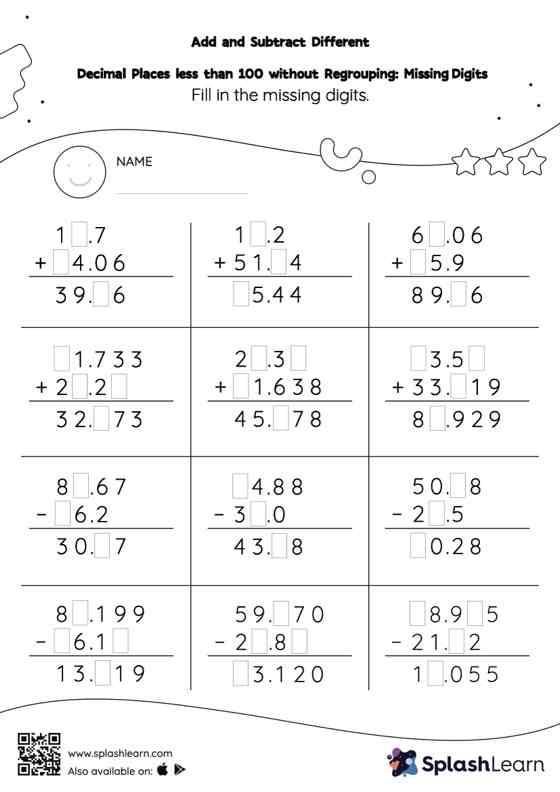# Add and Subtract Different Decimal Places less than 100 without Regrouping: Missing Digits Worksheet

Home > Add and Subtract Different Decimal Places less than 100 without Regrouping: Missing DigitsThis worksheet strengthens students' accuracy and strategic flexibility while they add and subtract different decimal places less than 100 without regrouping. Students find the missing number while adding and subtracting decimals by using the relationship between addition and subtraction. Add and subtract different decimal places less than 100 without regrouping worksheet does not require them to regroup numbers. This worksheet is about practicing the column method in which numbers are written one on top of another. In this method, students use their place value understanding to solve the problems.